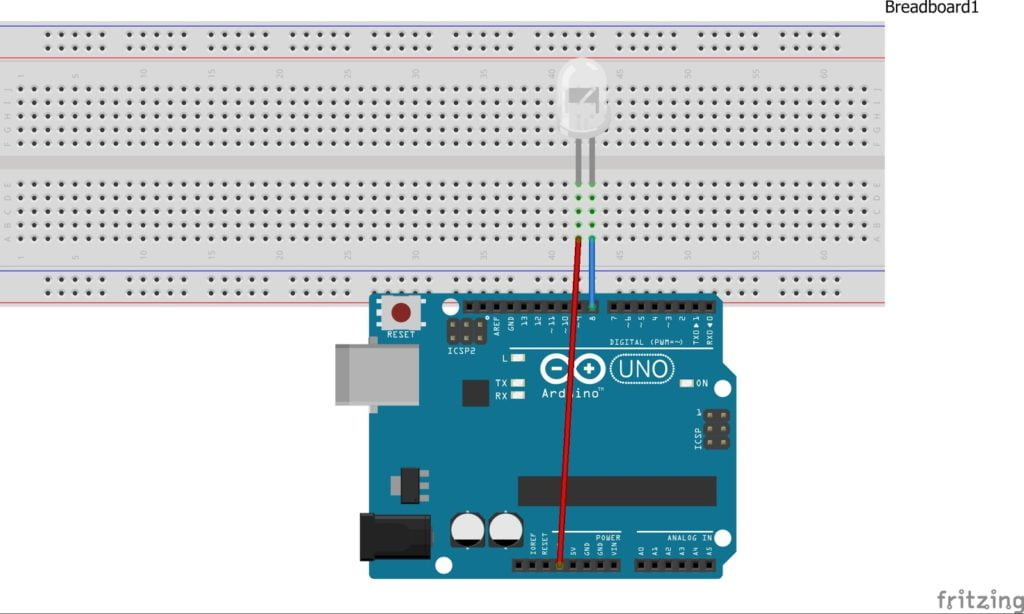## Interface LED with Arduino

This is the first tutorial in Arduino section, today we are going to light up an LED using Arduino so let’s get started.

## Thing required:

1.Arduino UNO or any other Arduino board.
3.Some jumper wires
4.A LED and a corresponding resistor.

## Connections

connect the circut as per the circuit diagram is shown. connect the LEDs positive leg to 3.3v on Arduino and connect the LEDs negative leg to pin 8 on Arduino. Here we are switching the negative leg of the LED if we want to switch the positive leg the negative of the LED will be connected to the GND(ground) of the Arduino and positive will be connected to the 8th pin of Arduino and there will be a small change in the program also.

×